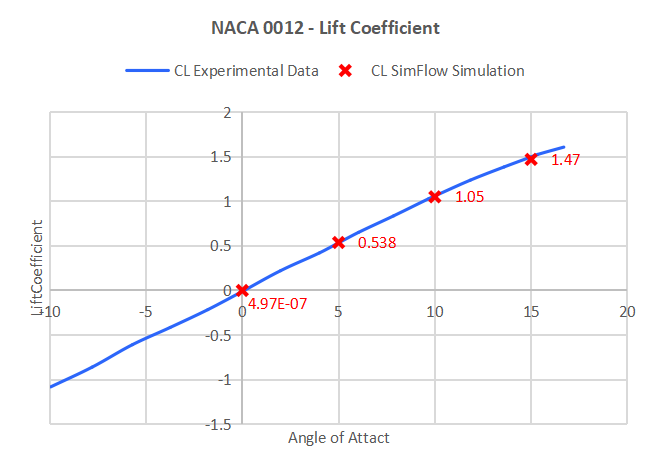Back to all tutorials

# Airfoil (NACA 0012) - CFD Simulation SimFlow Tutorial

## 1. Introduction

Airfoil NACA 0012 tutorial presents the analysis of the airfoil profile. Using an additional feature dedicated to 2D airfoil meshing and analysis you will be able to perform such a simulation in the shortest way.

SimFlow is a general purpose CFD Software

## 3. Enable Airfoil Feature

We need to enable the Airfoil feature in the SimFlow launcher in the Advanced Settings section.

1. Go to panel
2. Expand features list by clicking Manage Features
3. Choose Airfoil from the list and close the Advanced Settings panel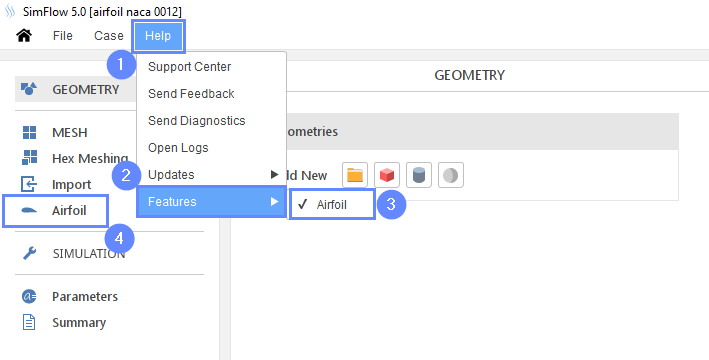## 4. Create Case

Open SimFlow and create a new case named airfoil_naca_0012

1. Go to panel
2. Provide name airfoil_naca_0012
3. Click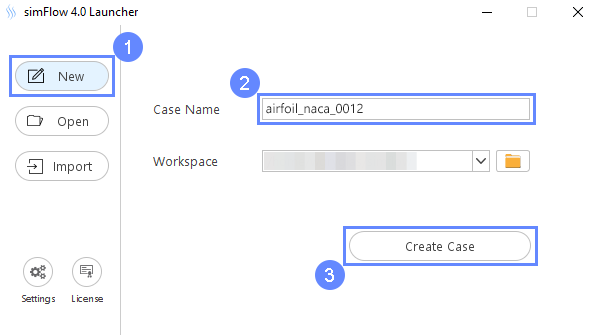## 5. Import Geometry

1. Go to Airfoil panel
2. Click
3. Select geometry file naca0012.dat
4. Click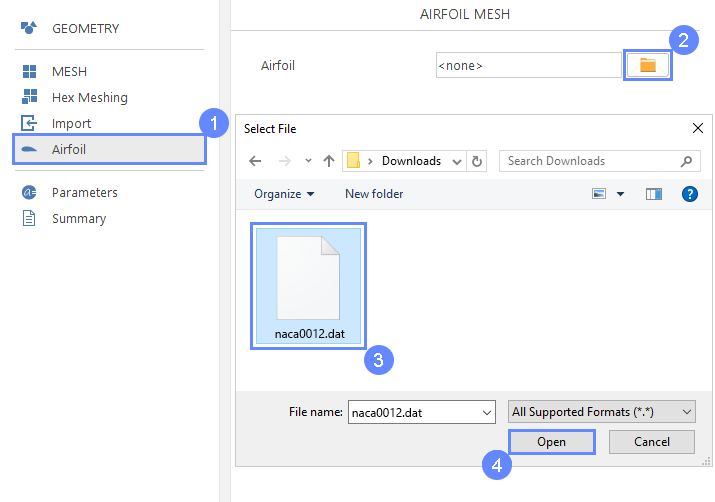## 6. Airfoil Meshing

1. Set Radius [m] to 25 meters
2. Set the following parameters accordingly
Surface Cell Thickness $${\sf [m]}$$2e-04
Min Surface Cell Length $${\sf [m]}$$2e-03
Max Surface Cell Length $${\sf [m]}$$8e-03
3. Click to start the meshing process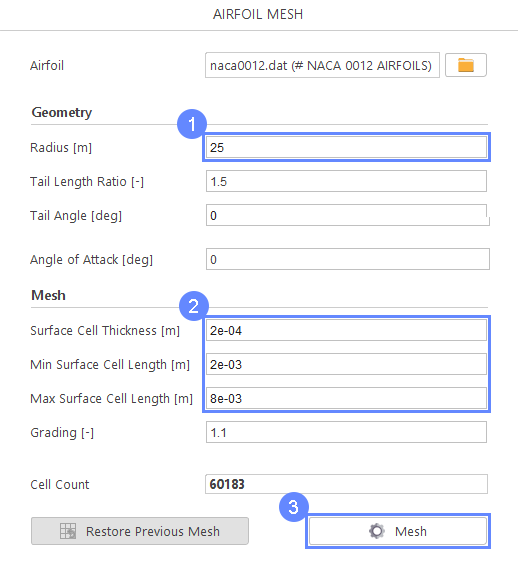## 7. Mesh

After creating a mesh, it will appear in the 3D window.

1. Click
2. Click to zoom the mesh
3. Change view projection from Perspective to
4. Zoom in the middle section of the mesh by using scroll mouse button
5. If you zoom in enough, airfoil shape should appear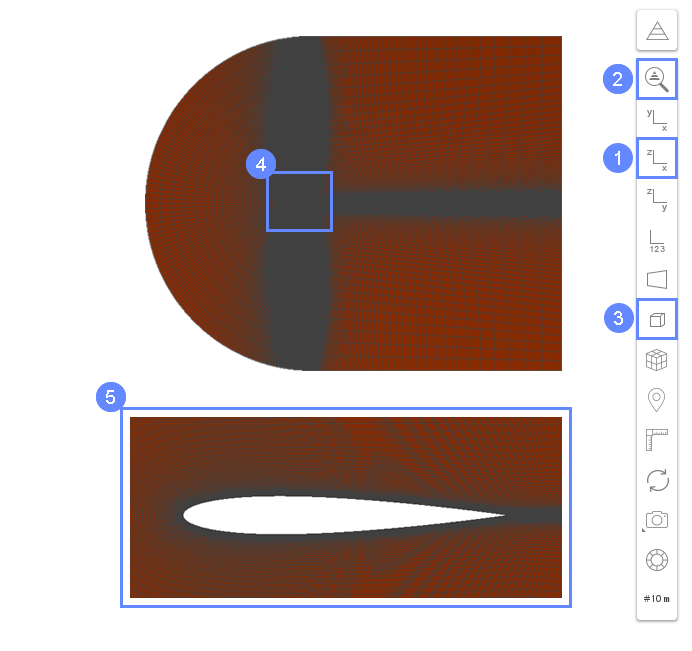## 8. Inspect Mesh Boundaries

Now we will check if the boundaries are set properly.

1. Inspect boundaries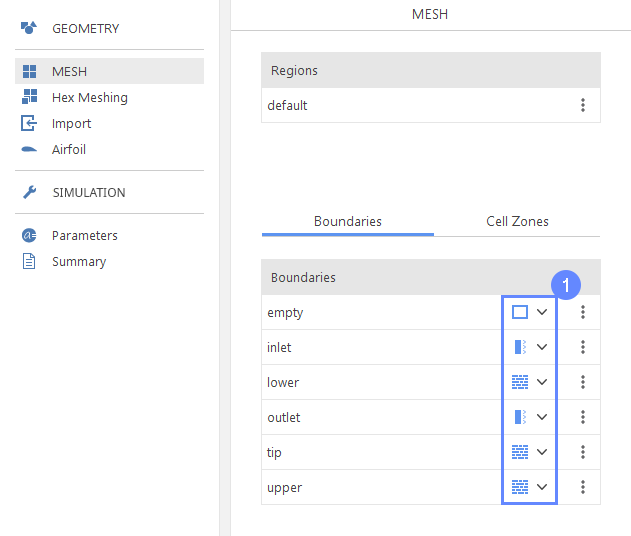## 9. Select Solver - SIMPLE

We want to analyze incompressible turbulent flow around the Airfoil. For this purpose, we will use the SIMPLE (simpleFoam) solver.

1. Go to Setup panel
2. Select filter
3. Select filter
4. Pick SIMPLE (simpleFoam) solver
5. solver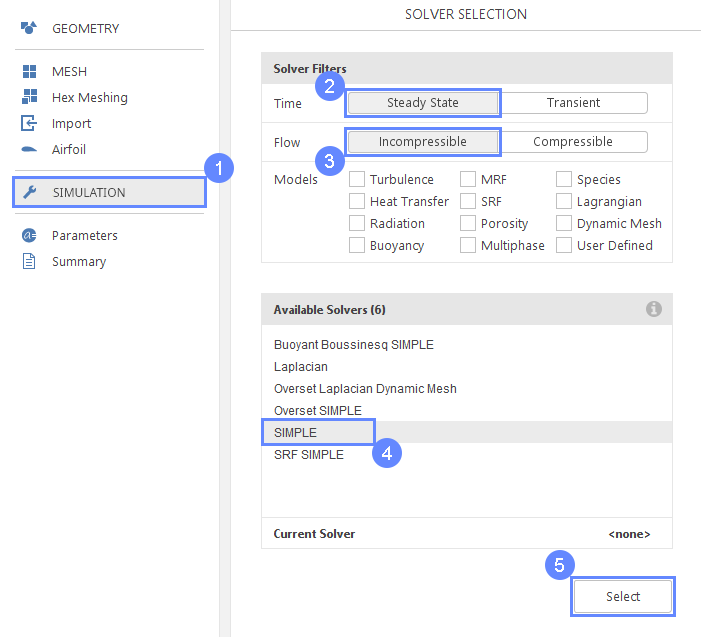## 10. Turbulence

We are going to use the standard $$k-\omega \; SST$$ model to handle turbulence. This model gives very good agreement with experimental data and is commonly used for aerodynamics applications.

1. Go to Turbulence panel
2. Select RANS modeling
3. Select $$k-\omega \; SST$$ model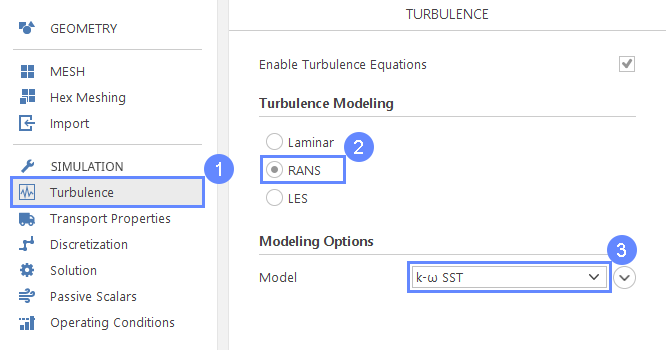## 11. Dicretization - Convection

1. Go to Discretization panel
2. Go to Convection tab
3. Select for U (Velocity) parameter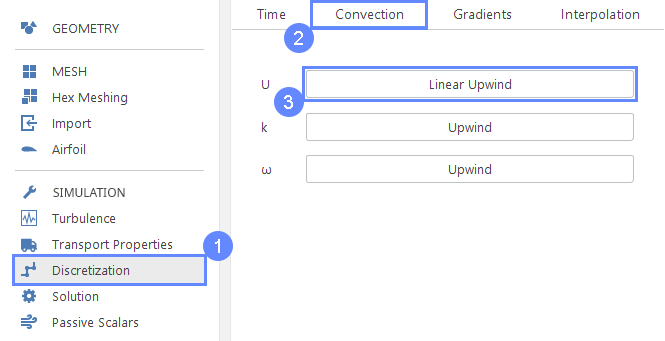## 12. Solution - Solvers (Pressure)

The calculations will be run until Residuals will drop down under 10 −6 . This criterion will guarantee high accuracy and will require more iteration. To make sure that the fluid solver will be able to capture very small changes in the flow, we need to make sure that the linear solvers for fluid flow equations will also be able to operate on very slight flow changes. To do this, we will change default solver tolerances.

1. Go to Solution panel
2. Expand list of options
3. Set Tolerance to 1e-07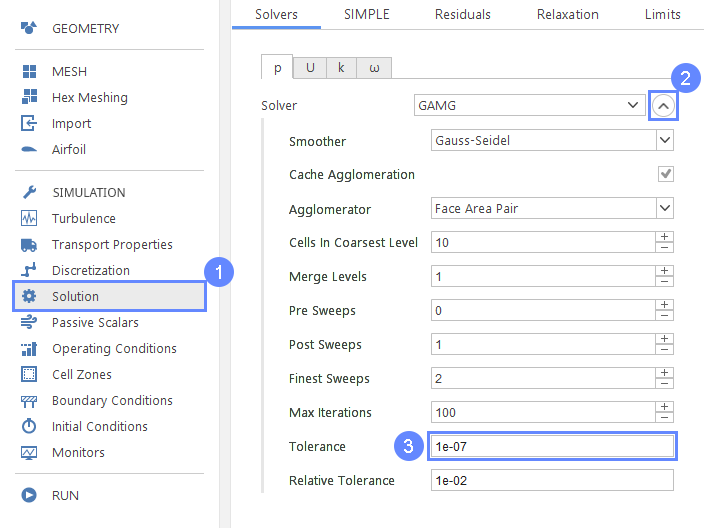## 13. Solution - Solvers (Velocity)

1. Go to U (Velocity) tab
2. Expand list of options
3. Set following parameters accordingly
Tolerance1e-07
Relative Tolerance1e-02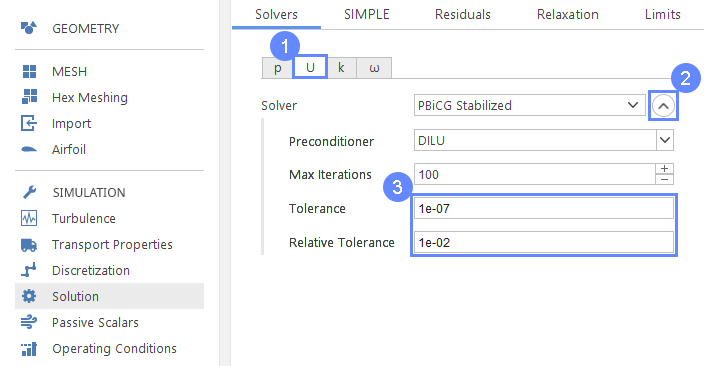## 14. Solution - SIMPLE

1. Go to SIMPLE tab
2. Set Non-Orthogonal Corrections to 2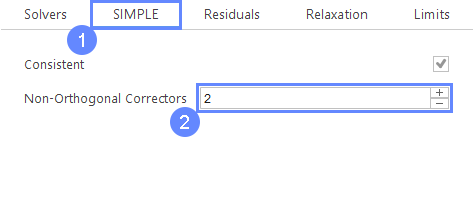## 15. Solution - Residuals

1. Go to Residuals tab
2. Set following parameters accordingly
p1e-06
U1e-06
k1e-05
$$\omega$$1e-05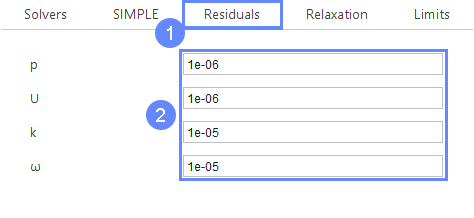## 16. Parameter - U (Velocity)

It is handy to parameterize velocity value to be easily accessible in the further setup. To be able to compare the results with test results we should assume $$Re=6000000$$. Based on the air viscosity $$\nu = 1.5 \cdot 10^{-5} \; m^2/s$$ and chord $$c\approx 1 \;m$$ we calculate the velocity from the equation: $$V=Re \cdot \frac{\nu}{c}$$

1. Go to Parameters panel
2. Define the name and formula of the new parameter
NameU
Formula6e6*(1.5e-5/1)
3. Click
4. The newly created parameter will be shown in the parameters list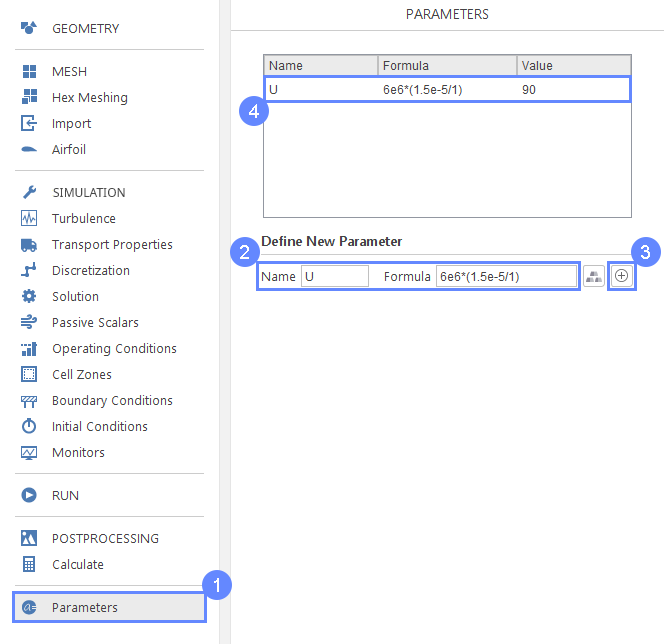## 17. Boundary Conditions - Inlet (Flow)

1. Go to Boundary Conditions panel
2. Select inlet boudary
3. Change boundary character to Free Stream
4. Set to velocity type to Free Stream
5. Now we can use parameter U defined earlier
Freestream Value $${\sf [m/s]}$$U00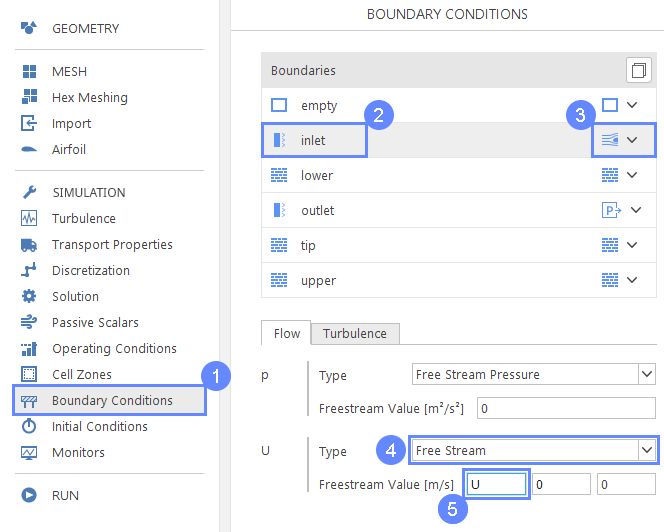## 18. Boundary Conditions - Inlet (Turbulence)

1. Go to Turbulence tab
2. Set Mixing Length $${\sf [m]}$$0.07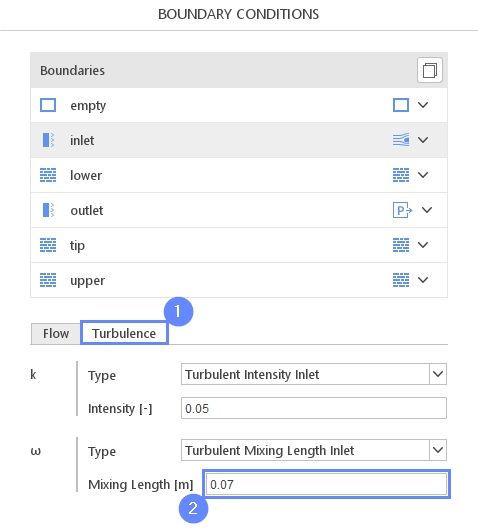## 19. Initial Conditions - Basic

1. Go to Initial Conditions panel
2. Set following initial conditions accordingly
UU00
k0.1
$$\omega$$100
$$\nu_t$$0.1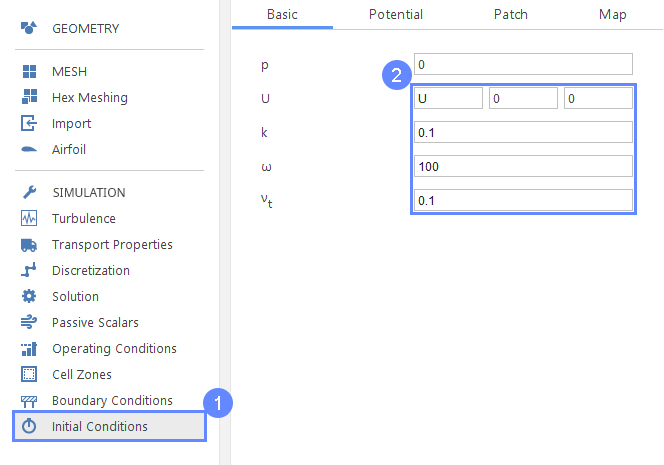## 20. Initial Conditions - Potential

We will use the "Potential" initialization feature. This utility solves pseudo potential flow prior to actual calculations. This will give a better initial guess for velocity and pressure fields.

1. Go to Potential tab
2. Check Initialize Potential Flow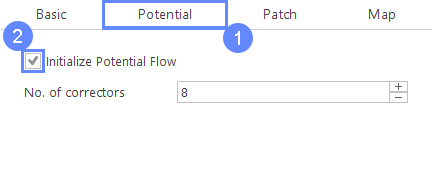## 21. Monitors - Forces

1. Go to the Monitors panel
2. Go to the Forces tab
3. For Monitor Boundaries select lower tip upper
4. Check Monitor Coefficients
5. Define free stram velocity accordingly

$$U_\infty$$ $${\sf [m/s]}$$U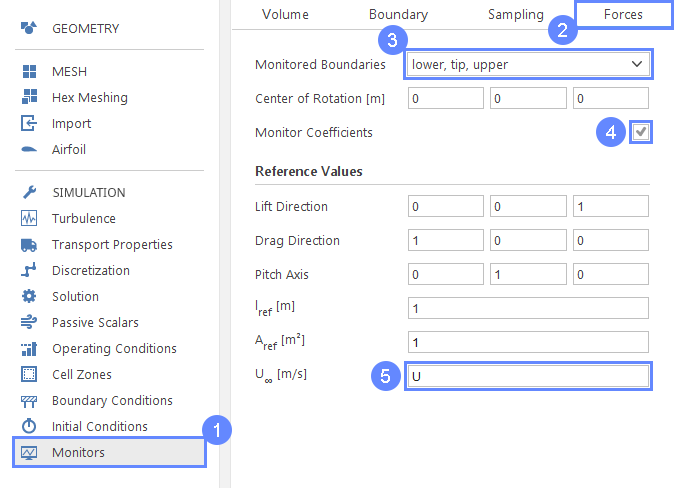## 22. Monitors - Create Slice

Additionally to observing force coefficients, we will display intermediate results on a section plane.

1. Go to Sampling tab
3. Set the follwing parameters accordingly
Normal $${\sf [-]}$$010
Point $${\sf [m]}$$000
4. Expand Fields menu. Choose p U k (pressure, velocity and turbulent kinetic energy) to be sampled on the section plane.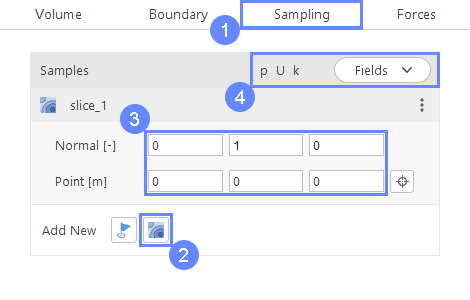## 23. Run - Time Control

1. Go to Run panel
2. Set Number of Iterations to 5000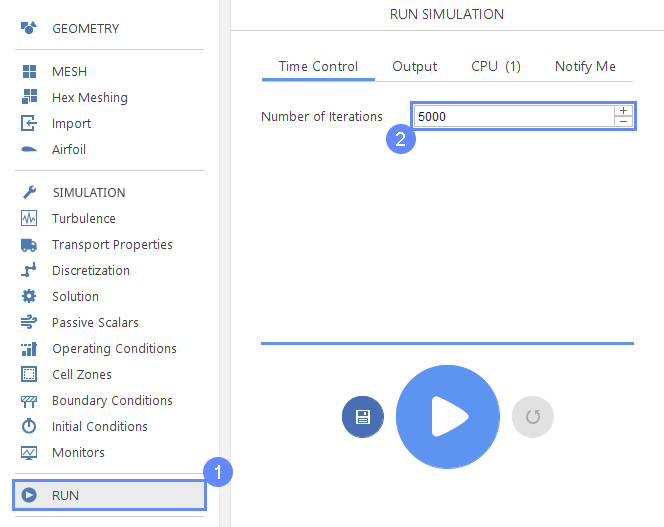## 24. Run - Output

1. Go to Output tab
2. Set Interval [-] to 100

This controls when results are written to the hard drive. When slices are enabled this also applies to them. Each new result will be displayed at a specified interval.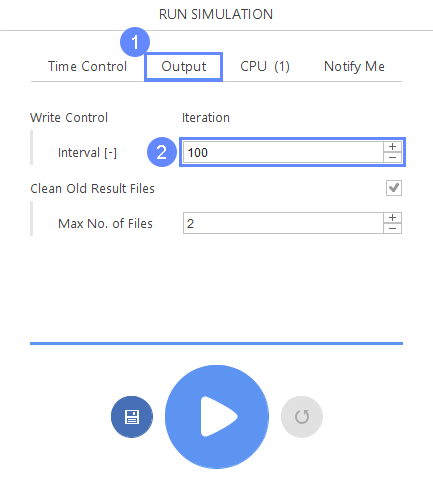## 25. Run - CPU

To speed up the calculation process increase the number of CPUs basing on your PC capability. The free version allows you to use only 2 processors in parallel mode. To get the full version, you can use the contact form to Request 30-day Trial

Estimated computation time for 2 processors: 15 minutes

1. Switch to CPU tab
2. Use parallel mode
3. Increase the Number of processors
4. Click button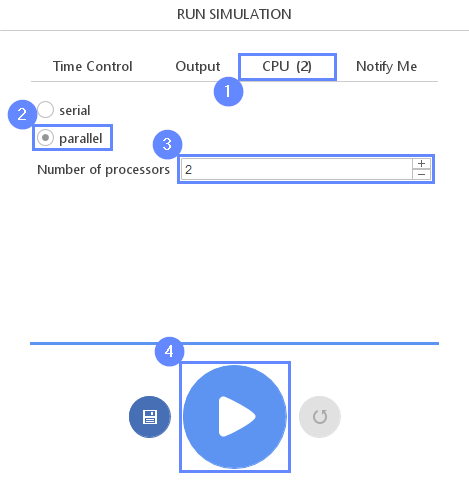## 26. Slice - View Velocity field

1. Change tab to Slices
2. Choose U (Velocity) field
3. Click Adjust range to data adjust color range to actually displayed data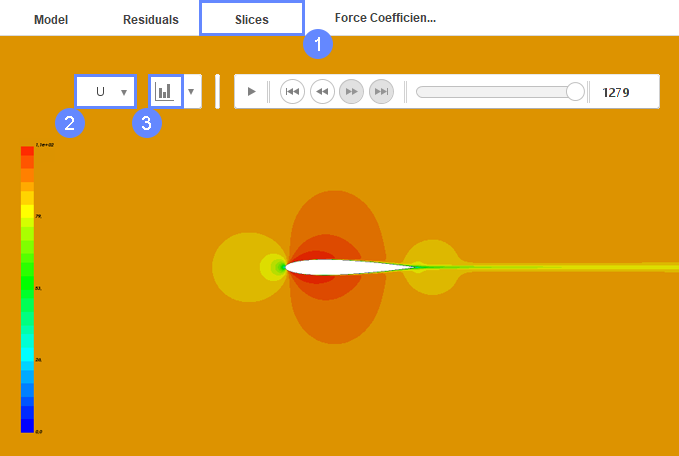## 27. Results - Force Coefficients

1. Change tab to Force Coefficients
2. Click Fit Axes
3. Select Lookup Data
4. Choose the last point from the chart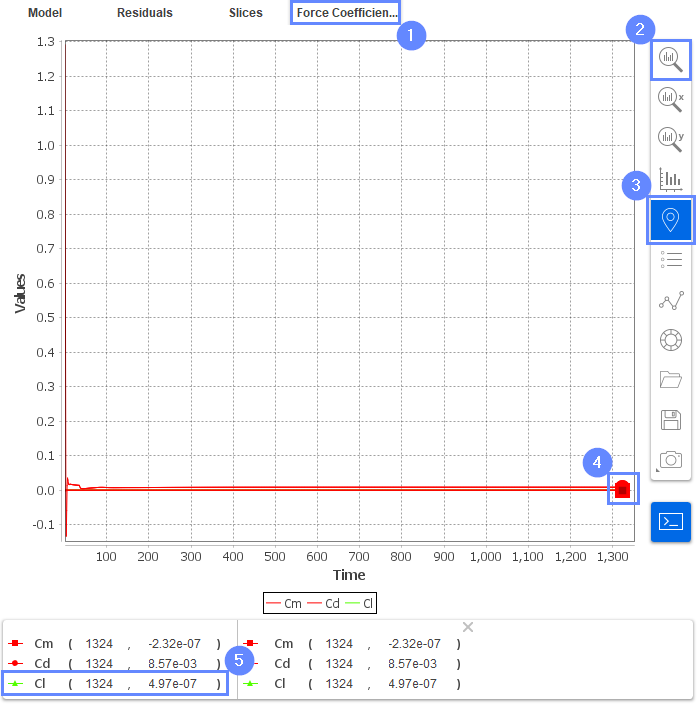## 28. Reset Simulation

If you want to change the angle of the attack, you should first reset the simulation in the run panel.

1. Go to Run Panel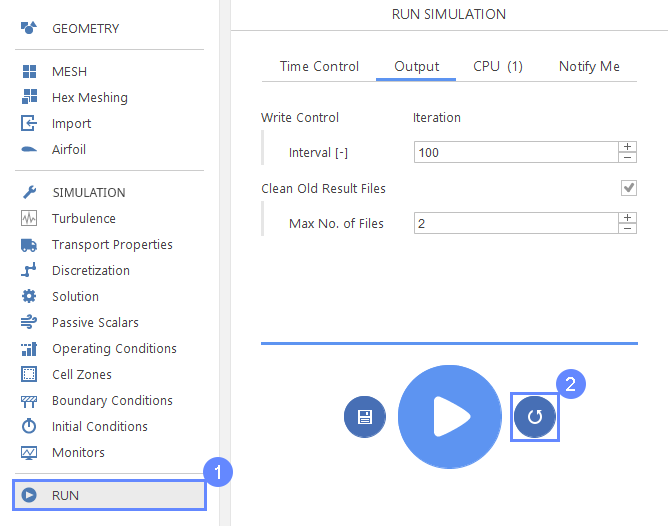## 29. Change Angle of Attack

In the Airfoil panel, we can now remesh the Airfoil change angle of attack. And then rerun the simulation.

1. Go to Airfoil panel
2. Change the Angle of attack for the desired value for example
Angle of Attack $${\sf [deg]}$$5
3. Click button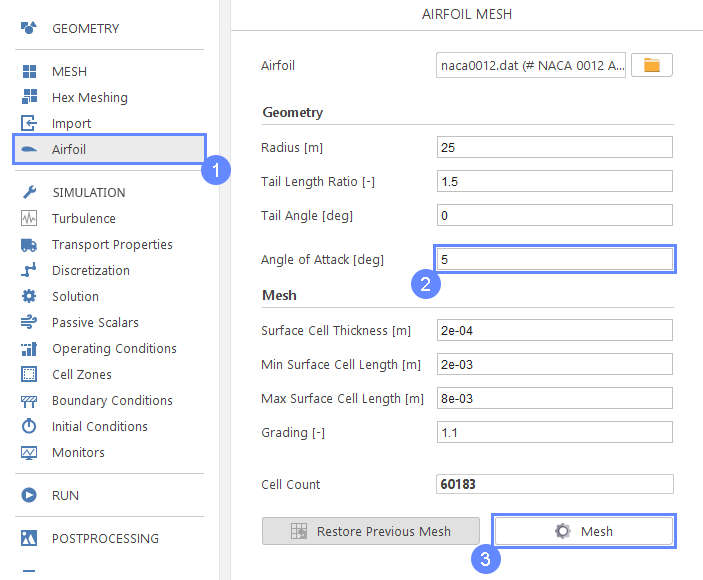## 30. Mesh - Second Case

After creating a mesh, it will appear in the 3D window.

1. Click XZ View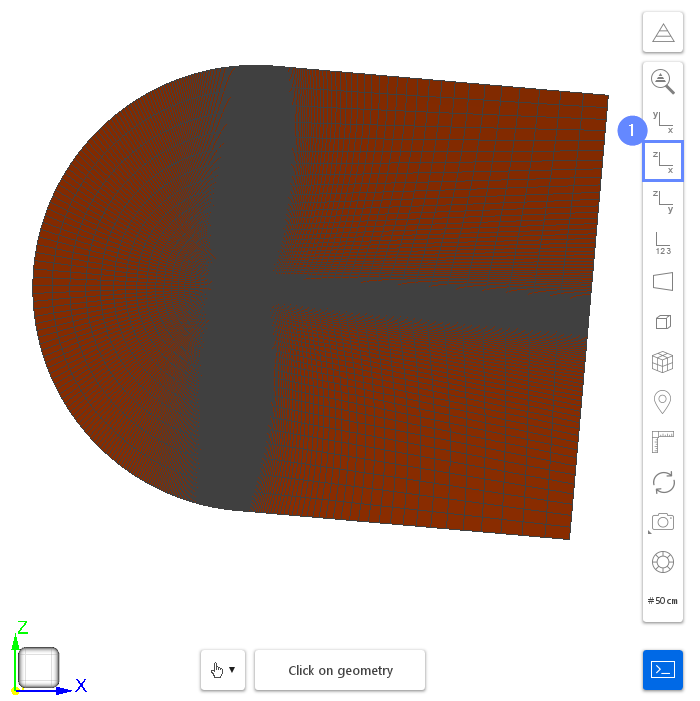## 31. Run Second Simulation

1. Go to Run panel
2. Click button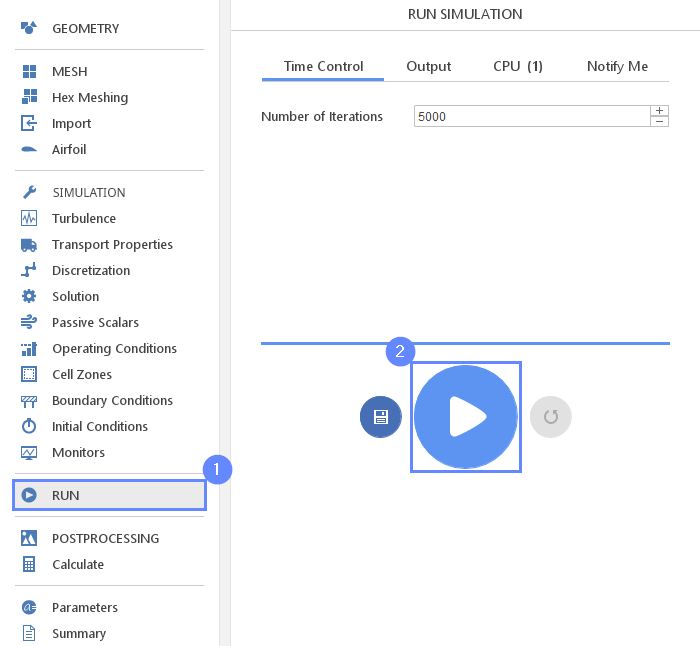## 32. Results Comparison With Experimental Data

You might repeat the above operations for different angles of attack, e.g: 5, 10 and 15 degrees. Next comparing them with the experimental data (NASA Langley, 1988) you should be able to obtain the following results.